Triangles to print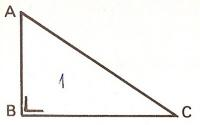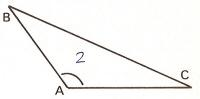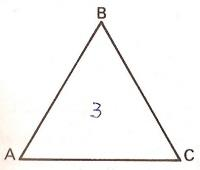Name: ______________________________________   Subject: _______________________  Date: _______

Write the missing word

1. Types of triangles based on angles

A triangle is a polygon with three sides and three interior angles.

If you see the triangle 1, you will see that it has a right angle (ABC), it is called right triangle. The triangle 2 has an obtuse angle (BAC), it is called obtuse triangle. The triangle 3 has 3 acute angles and it is called acute triangle.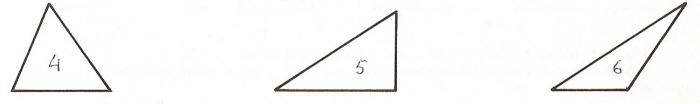The triangle 4 is... The triangle 5 is... The triangle 6 is...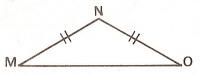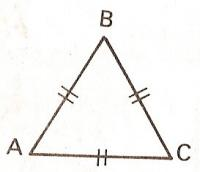2. Types of triangles based on sides

The triangle MNO has two equal sides (MN and NO) and another side that is greater (MO). The triangles with two equal sides are called isosceles. For example: MNO.

The triangle ABC has the three equal sides: AB = BC = CA. The triangles with three equal sides are called Equilateral. For example: ABC.

The triangle PQR has 3 unequal sides and it is called scalene.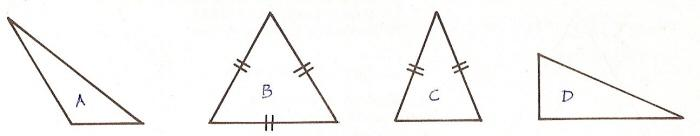Look at these pictures and answer these questions:

 The triangle A is... The triangle B is...... The triangle C is...... The triangle D is...3. Angle Sum of a triangle
1. In the triangle CAB, we can see the point M between points C and A. We can also note the point N between points A and B.
2. If we fold the paper in the line MN, the angle A stays down. We may fold in the red line from the point M and also from the point N.
3. We can see that the angle C plus A plus B is a straight angle. Thus, A + B + C = 180°.
The sum of the three angles of a triangle is a straight or 180 degree angle.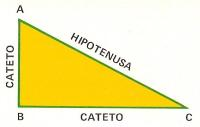4. The right triangle
A set-square has the form of a triangle and one side is right. That is why we call it right triangle. The side opposite of the right angle is called hypotenuse and the sides adjacent to the right angle are called legs.Look at this picture and answer these questions:

 The side MO is... The side MN is... The side ON is...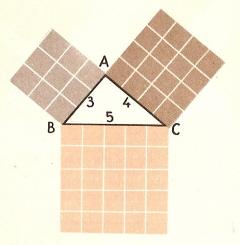5. The Pythagorean Theorem

The triangle ABC is a right triangle and we see that the leg AB is 3 cm, the leg AC is 4 cm and the hypotenuse BC is 5 cm. Then we square the 3 sides. The square of the hypotenuse BC is 52= 25 cm2, the square on the leg AC is 42 = 16 cm2 and the square on the leg AB is 32 = 9 cm2.

Note that 25=16 + 9.

The square of the hypotenuse of a right triangle is equal to the sum of the squares of the legs.

Pythagoras was a Greek mathematician. He was born in Samos in 580 BC. He learned from the Egyptians the relationship between the hypotenuse and the legs and generalized the famous theorem.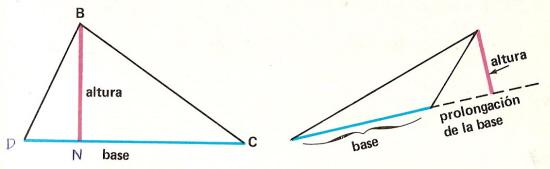;

6. Triangle parts
l    If we have a triangle DBC and we draw a perpendicular next to DC from the vertex B, we have the altitude. In this case, the DC side is called the base.
On the other obtuse triangle, the altitude is on the base extension.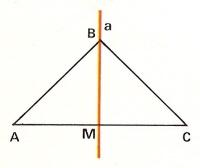Look at the picture and answer these questions:

 The side AB is the base: The side AC is the base: The side BC is the altitude: The side BM is the altitude: The side AM is the base: The side MC is the base:

| Educational applications | Mathematics |
In Spanish  | Interactive

®Arturo Ramo García.-Record of intellectual property of Teruel (Spain) No 141, of 29-IX-1999
Plaza Playa de Aro, 3, 1ş DO 44002-TERUEL